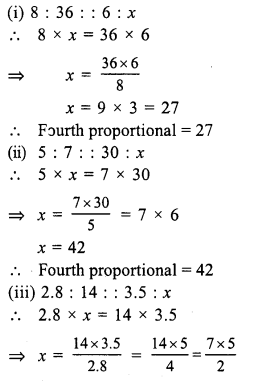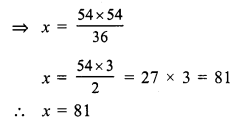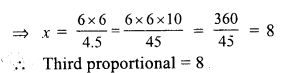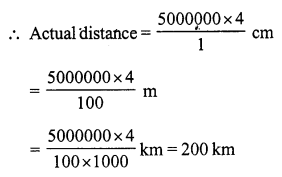## RS Aggarwal Class 7 Solutions Chapter 8 Ratio and Proportion Ex 8B

These Solutions are part of RS Aggarwal Solutions Class 7. Here we have given RS Aggarwal Solutions Class 7 Chapter 8 Ratio and Proportion Ex 8B.

Other Exercises

Question 1.
Solution:
We know that a, b, c, d are in proportion if ad = bc
Now 30, 40, 45, 60 are in proportion
if 30 x 60 = 40 x 45
if 1800= 1800
which is true
30, 40, 45, 60 are in proportion.

Question 2.
Solution:
We know that if a, b, c, d are in proportion if ad = bc
Now 36, 49, 6, 7 are in proportion
if 36 x 7 = 49 x 6
if 252 = 294
But 252 ≠ 294
36, 49, 6, 7 are not in proportion

Question 3.
Solution:
2 : 9 : : x : 27
9 x x = 2 x 27
x = $$\frac { 2 x 27 }{ 9 }$$ = 2 x 3 = 6

Question 4.
Solution:
8 : x : : 16 : 35
x x 16 = 8 x 35
x = $$\frac { 8 x 35 }{ 6 }$$ = $$\frac { 35 }{ 2 }$$ = 17.5

Question 5.
Solution:
x : 35 : : 48 : 60
x x 60 = 35 x 48
x = 7 x 4 = 28

Question 6.
Solution:
Let x be the fourth proportional, thenx = $$\frac { 35 }{ 2 }$$ = 17.5
Fourth proportional = 17.5

Question 7.
Solution:
36, 54, x are in continued proportion
36 : 54 : : 54 : x
⇒ 36 x x = 54 x 54Question 8.
Solution:
27, 36, x are in continued proportion
27 : 36 :: 36 : x
27 x x = 36 x 36Question 9.
Solution:
Let x be the third proportional, then
(i) 8 : 12 : : 12 : xQuestion 10.
Solution:
Third proportional = 28 then
7 : x :: x : 28
⇒ 7 x 28 = x x x
⇒ x2 = 28 x 7 = 196
⇒ x = √196 = 14
x = 14

Question 11.
Solution:
Let x be the mean proportional, then
(i) 6 : x :: x : 24
⇒ x2 = 6 x 24 = 144
x = √144 = 12
Mean proportional = 12
(ii) 3 : x : : x : 27
⇒ x2 = 3 x 27 = 81
x = √81 = 9
Mean proportional = 9
(iii) 0.4 : x :: x : 0.9
⇒ x2 = 0.4 x 0.9
x = √o.36 = 0.6
Mean proportional = 0.6

Question 12.
Solution:
Let x be added to each of the given numbers then
5 + x, 9 + x, 7 + x, 12 + x are in proportion
$$\frac { 5 + x }{ 9 + x }$$ = $$\frac { 7 + x }{ 12 + x }$$
By cross multiplication :
(5 + x) (12 + x) = (7 + x) (9 + x)
⇒ 60 + 5x + 12x + x2 = 63 + 7x + 9x + x2
⇒ 60 + 17x + x2 = 63 + 16x + x2
⇒ 17x + x2 – 16x – x2 = 63 – 60
⇒ x = 3
Required number = 3

Question 13.
Solution:
Let x be subtracted from each of the given number, then
10 – x, 12 – x, 19 – x and 24 – x are in proportion
$$\frac { 10 – x }{ 12 – x }$$ = $$\frac { 19 – x }{ 24 – x }$$
By cross multiplication :
(10 – x) (24 – x) = (19 – x) (12 – x)
⇒ 240 – 10x – 24x + x2 = 228 – 19x – 12x + x2
⇒ 240 – 34x + x2 = 228 – 31x + x2
⇒ -34x + x2 + 31x – x2 = 228 – 240
⇒ -3x = -2
⇒ 3x = 12
⇒ x = 4
Required number = 4

Question 14.
Solution:
Scale of map = 1 : 5000000
Distance between two town on the map = 4 cmQuestion 15.
Solution:
Height of a tree = 6 cm
and its shadow at same time = 8 m
Shadow of a pole = 20 m
Let height of pole = x m
6 : 8 = x : 20
⇒ x= $$\frac { 6 x 20 }{ 8 }$$ = 15 m
Height of pole = 15 m

Hope given RS Aggarwal Solutions Class 7 Chapter 8 Ratio and Proportion Ex 8B are helpful to complete your math homework.

If you have any doubts, please comment below. Learn Insta try to provide online math tutoring for you.